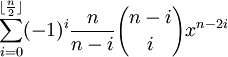## Saturday, February 03, 2007

### Van Roomen's Problem: Solution Explained

In today's blog, I will provide the solution to Van Roomen's problem. Van Roomen's problem established François Viète as one of the most important mathematicians of his day, showed the relevance of trigonometry to solving algebraic equations, and finally paved the way to Albert Girard's insight (and Carl Friedrich Gauss's brilliant proof) of the Fundamental Theorem of Algebra.

First, here is the problem posed by Adriaan Van Roomen (see here for more details):

Solve for x where:

(45)x - (3,795)x3 + (95,634)x5 - (1,138,500)x7 + (7,811,375)x9 - (34,512,075)x11 + (105,306,075)x13 - (232,676,280)x15 + (384,942,375)x17 - (488,494,125)x19 + (483,841,800)x21 - (378,658,800)x23 + (236,030,652)x25 - (117,679,100)x27 + (46,955,700)x29 - (14,945,040)x31 + (3,764,565)x33 - (740,259)x35 + (111,150)x37 - (12,300)x39 + (945)x41 - (45)x43 + x45 = A

and:Let's start by simplifying Van Roomen's equation.

If we define:

Fn(x) =Then, it turns out (see here for the details) that:

F45(x) =

(45)x - (3,795)x3 + (95,634)x5 - (1,138,500)x7 + (7,811,375)x9 - (34,512,075)x11 + (105,306,075)x13 - (232,676,280)x15 + (384,942,375)x17 - (488,494,125)x19 + (483,841,800)x21 - (378,658,800)x23 + (236,030,652)x25 - (117,679,100)x27 + (46,955,700)x29 - (14,945,040)x31 + (3,764,565)x33 - (740,259)x35 + (111,150)x37 - (12,300)x39 + (945)x41 - (45)x43 + x45

The complex value for A can also be simplified. It turns out that (see Lemma 7, here):= 2 sin(π/)

Now, analyzing the above equation for Fn, also establishes the following linkage to trigonometry (see Corollary 1.2, here for details):

For any odd integer n ≥ 1:

2 sin(nα) = (-1)(n-1)/2*Fn(2sinα)

So, for n = 45, we have:

2sin(45α) = (-1)22F45(2sinα) = F45(2sinα)

Now, we have enough to derive the solution that Van Roomen was interested in since:

2sin(45α) = F45(2sinα) = 2 sin(π/)

We just need to solve for α so that:

45α = π/15 so α = π/[15*45].

Thus, the solution is x = 2sinα = 2sin(π/[15*45])

Indeed, if we look at all of Van Roomen's examples that he gave (see here for details), the same solution applies (see here for the proofs). In each case, you will find that if A = 2 sin(θ), then x = 2sin(θ/45).

This solution was enough for Van Roomen but this was not enough for Viète. He went on to show that there are 45 different solutions for x (44 in addition to the one listed above) with 23 positive solutions and 22 negative solutions. This insight would be very important to establishing the Fundamental Theorem of Algebra.

How did he do it?

Well, we know that for sin, sin(θ) = sin(θ + 2π). [See Property 5, here for details if needed]

This means that if we define αk = α + n*(2π/45), then in all cases:

2sin(45*[α + n*(2π/45)]) = 2sin(π/15).

We know that there are 45 solutions because (n*2π/45) for n =0, ..., 44 are all distinct values. At n=45, 45*2π/45 = 2π which is the same value as n=0 since sine is a repeating function and so on for any value n ≥ 45.

We know that for n= 0, ..., 22, sin(α + n*(2π/45)) ≥ 0 and for n = 23, ..., 44 sin(α + n*(2π/45)) is negative.

In my next blog, I will show how François Viète came up with his own solution to the cubic equation.

References
• Jean-Pierre Tignol, Galois' Theory of Algebraic Equations, World Scientific, 2001